# Mean State

Period Mean (original grids) [Watt m-2]
Model Period Mean (intersection) [Watt m-2]
Model Period Mean (complement) [Watt m-2]
Benchmark Period Mean (intersection) [Watt m-2]
Benchmark Period Mean (complement) [Watt m-2]
Bias [Watt m-2]
RMSE [Watt m-2]
Phase Shift [months]
Bias Score 
RMSE Score 
Seasonal Cycle Score 
Spatial Distribution Score 
Interannual Variability Score 
Overall Score 
Benchmark [-] 217.
CRUNCEPv7 [-] 228. 227. 217. 232. 11.0 22.1 0.909 0.580 0.471 0.873 0.992 0.520 0.651
GSWP3v1 [-] 217. 216. 217. 232. 0.504 14.1 0.318 0.860 0.543 0.956 0.999 0.759 0.777
WFDEI [-] 199. 198. 217. 232. -17.3 30.3 1.03 0.443 0.421 0.838 0.859 0.724 0.617
Period Mean (original grids) [Watt m-2]
Model Period Mean (intersection) [Watt m-2]
Model Period Mean (complement) [Watt m-2]
Benchmark Period Mean (intersection) [Watt m-2]
Benchmark Period Mean (complement) [Watt m-2]
Bias [Watt m-2]
RMSE [Watt m-2]
Phase Shift [months]
Bias Score 
RMSE Score 
Seasonal Cycle Score 
Spatial Distribution Score 
Interannual Variability Score 
Overall Score 
Benchmark [-] 241.
CRUNCEPv7 [-] 245. 245. 241. 238. 4.28 22.8 0.581 0.648 0.524 0.951 0.929 0.663 0.706
GSWP3v1 [-] 240. 241. 241. 238. -0.171 13.3 0.143 0.901 0.613 0.990 0.999 0.815 0.822
WFDEI [-] 236. 237. 241. 238. -4.14 24.3 0.456 0.649 0.501 0.959 0.755 0.772 0.689
Period Mean (original grids) [Watt m-2]
Model Period Mean (intersection) [Watt m-2]
Model Period Mean (complement) [Watt m-2]
Benchmark Period Mean (intersection) [Watt m-2]
Benchmark Period Mean (complement) [Watt m-2]
Bias [Watt m-2]
RMSE [Watt m-2]
Phase Shift [months]
Bias Score 
RMSE Score 
Seasonal Cycle Score 
Spatial Distribution Score 
Interannual Variability Score 
Overall Score 
Benchmark [-] 233.
CRUNCEPv7 [-] 231. 231. 233. 238. -1.27 21.2 0.855 0.727 0.552 0.892 0.906 0.548 0.696
GSWP3v1 [-] 233. 233. 233. 238. 0.143 15.9 0.237 0.890 0.601 0.969 0.994 0.696 0.792
WFDEI [-] 233. 233. 233. 238. -0.0886 23.3 0.790 0.765 0.510 0.900 0.950 0.669 0.717
Period Mean (original grids) [Watt m-2]
Model Period Mean (intersection) [Watt m-2]
Model Period Mean (complement) [Watt m-2]
Benchmark Period Mean (intersection) [Watt m-2]
Benchmark Period Mean (complement) [Watt m-2]
Bias [Watt m-2]
RMSE [Watt m-2]
Phase Shift [months]
Bias Score 
RMSE Score 
Seasonal Cycle Score 
Spatial Distribution Score 
Interannual Variability Score 
Overall Score 
Benchmark [-] 111.
CRUNCEPv7 [-] 112. 112. 111. 117. 0.518 16.3 0.0404 0.936 0.826 0.997 0.992 0.507 0.847
GSWP3v1 [-] 107. 107. 111. 117. -3.90 12.6 0.0241 0.948 0.850 0.998 0.896 0.691 0.872
WFDEI [-] 102. 102. 111. 117. -9.10 21.4 0.608 0.899 0.780 0.960 0.921 0.741 0.847
Period Mean (original grids) [Watt m-2]
Model Period Mean (intersection) [Watt m-2]
Model Period Mean (complement) [Watt m-2]
Benchmark Period Mean (intersection) [Watt m-2]
Benchmark Period Mean (complement) [Watt m-2]
Bias [Watt m-2]
RMSE [Watt m-2]
Phase Shift [months]
Bias Score 
RMSE Score 
Seasonal Cycle Score 
Spatial Distribution Score 
Interannual Variability Score 
Overall Score 
Benchmark [-] 213.
CRUNCEPv7 [-] 216. 215. 209. 225. 9.33 24.3 1.51 0.558 0.429 0.778 0.905 0.494 0.599
GSWP3v1 [-] 205. 205. 209. 225. 0.417 17.6 0.524 0.789 0.464 0.924 0.989 0.769 0.733
WFDEI [-] 190. 190. 209. 225. -14.3 27.6 1.23 0.501 0.408 0.813 0.963 0.628 0.620
Period Mean (original grids) [Watt m-2]
Model Period Mean (intersection) [Watt m-2]
Model Period Mean (complement) [Watt m-2]
Benchmark Period Mean (intersection) [Watt m-2]
Benchmark Period Mean (complement) [Watt m-2]
Bias [Watt m-2]
RMSE [Watt m-2]
Phase Shift [months]
Bias Score 
RMSE Score 
Seasonal Cycle Score 
Spatial Distribution Score 
Interannual Variability Score 
Overall Score 
Benchmark [-] 126.
CRUNCEPv7 [-] 134. 134. 126. 145. 7.98 18.7 0.157 0.891 0.817 0.989 0.996 0.577 0.848
GSWP3v1 [-] 126. 126. 126. 145. 0.0721 11.8 0.0425 0.982 0.860 0.997 1.00 0.637 0.889
WFDEI [-] 119. 118. 126. 145. -6.86 18.3 0.258 0.910 0.811 0.983 0.954 0.741 0.868
Period Mean (original grids) [Watt m-2]
Model Period Mean (intersection) [Watt m-2]
Model Period Mean (complement) [Watt m-2]
Benchmark Period Mean (intersection) [Watt m-2]
Benchmark Period Mean (complement) [Watt m-2]
Bias [Watt m-2]
RMSE [Watt m-2]
Phase Shift [months]
Bias Score 
RMSE Score 
Seasonal Cycle Score 
Spatial Distribution Score 
Interannual Variability Score 
Overall Score 
Benchmark [-] 170.
CRUNCEPv7 [-] 188. 187. 170. 169. 16.8 27.8 0.502 0.723 0.736 0.959 0.893 0.618 0.777
GSWP3v1 [-] 171. 171. 170. 169. 0.441 14.1 0.129 0.949 0.787 0.988 0.999 0.620 0.855
WFDEI [-] 180. 179. 170. 169. 8.92 24.9 0.541 0.815 0.696 0.952 0.949 0.691 0.800
Period Mean (original grids) [Watt m-2]
Model Period Mean (intersection) [Watt m-2]
Model Period Mean (complement) [Watt m-2]
Benchmark Period Mean (intersection) [Watt m-2]
Benchmark Period Mean (complement) [Watt m-2]
Bias [Watt m-2]
RMSE [Watt m-2]
Phase Shift [months]
Bias Score 
RMSE Score 
Seasonal Cycle Score 
Spatial Distribution Score 
Interannual Variability Score 
Overall Score 
Benchmark [-] 210.
CRUNCEPv7 [-] 231. 231. 209. 224. 21.5 32.3 0.302 0.561 0.586 0.978 0.990 0.539 0.707
GSWP3v1 [-] 208. 208. 209. 224. 0.482 16.5 0.148 0.894 0.657 0.990 0.991 0.664 0.809
WFDEI [-] 207. 207. 209. 224. -1.19 28.0 0.414 0.698 0.550 0.970 0.817 0.650 0.706
Period Mean (original grids) [Watt m-2]
Model Period Mean (intersection) [Watt m-2]
Model Period Mean (complement) [Watt m-2]
Benchmark Period Mean (intersection) [Watt m-2]
Benchmark Period Mean (complement) [Watt m-2]
Bias [Watt m-2]
RMSE [Watt m-2]
Phase Shift [months]
Bias Score 
RMSE Score 
Seasonal Cycle Score 
Spatial Distribution Score 
Interannual Variability Score 
Overall Score 
Benchmark [-] 105.
CRUNCEPv7 [-] 103. 104. 105. 108. -1.87 18.0 0.284 0.918 0.796 0.981 0.901 0.517 0.818
GSWP3v1 [-] 96.3 96.5 105. 108. -8.76 12.5 0.0632 0.901 0.827 0.996 0.798 0.644 0.832
WFDEI [-] 89.7 89.9 105. 108. -15.3 20.2 0.176 0.840 0.782 0.988 0.975 0.698 0.844
Period Mean (original grids) [Watt m-2]
Model Period Mean (intersection) [Watt m-2]
Model Period Mean (complement) [Watt m-2]
Benchmark Period Mean (intersection) [Watt m-2]
Benchmark Period Mean (complement) [Watt m-2]
Bias [Watt m-2]
RMSE [Watt m-2]
Phase Shift [months]
Bias Score 
RMSE Score 
Seasonal Cycle Score 
Spatial Distribution Score 
Interannual Variability Score 
Overall Score 
Benchmark [-] 228.
CRUNCEPv7 [-] 235. 235. 229. 216. 2.90 18.7 0.429 0.877 0.736 0.971 0.970 0.654 0.824
GSWP3v1 [-] 232. 232. 229. 216. 0.0913 15.0 0.0879 0.946 0.762 0.993 1.00 0.677 0.857
WFDEI [-] 237. 237. 229. 216. 4.10 24.4 0.294 0.882 0.654 0.979 1.00 0.799 0.828
Period Mean (original grids) [Watt m-2]
Model Period Mean (intersection) [Watt m-2]
Model Period Mean (complement) [Watt m-2]
Benchmark Period Mean (intersection) [Watt m-2]
Benchmark Period Mean (complement) [Watt m-2]
Bias [Watt m-2]
RMSE [Watt m-2]
Phase Shift [months]
Bias Score 
RMSE Score 
Seasonal Cycle Score 
Spatial Distribution Score 
Interannual Variability Score 
Overall Score 
Benchmark [-] 140.
CRUNCEPv7 [-] 158. 158. 138. 161. 20.2 28.5 0.194 0.752 0.799 0.987 0.997 0.642 0.829
GSWP3v1 [-] 135. 135. 138. 161. 0.0454 13.3 0.0808 0.952 0.846 0.994 0.998 0.641 0.880
WFDEI [-] 136. 136. 138. 161. 0.763 21.0 0.264 0.905 0.777 0.982 0.977 0.770 0.865
Period Mean (original grids) [Watt m-2]
Model Period Mean (intersection) [Watt m-2]
Model Period Mean (complement) [Watt m-2]
Benchmark Period Mean (intersection) [Watt m-2]
Benchmark Period Mean (complement) [Watt m-2]
Bias [Watt m-2]
RMSE [Watt m-2]
Phase Shift [months]
Bias Score 
RMSE Score 
Seasonal Cycle Score 
Spatial Distribution Score 
Interannual Variability Score 
Overall Score 
Benchmark [-] 191.
CRUNCEPv7 [-] 191. 191. 186. 193. 4.01 23.2 0.455 0.761 0.685 0.952 0.984 0.544 0.769
GSWP3v1 [-] 182. 182. 186. 193. -4.43 14.4 0.132 0.905 0.731 0.986 0.986 0.662 0.833
WFDEI [-] 184. 184. 186. 193. -3.04 24.7 0.454 0.773 0.654 0.953 0.981 0.695 0.785
Period Mean (original grids) [Watt m-2]
Model Period Mean (intersection) [Watt m-2]
Model Period Mean (complement) [Watt m-2]
Benchmark Period Mean (intersection) [Watt m-2]
Benchmark Period Mean (complement) [Watt m-2]
Bias [Watt m-2]
RMSE [Watt m-2]
Phase Shift [months]
Bias Score 
RMSE Score 
Seasonal Cycle Score 
Spatial Distribution Score 
Interannual Variability Score 
Overall Score 
Benchmark [-] 240.
CRUNCEPv7 [-] 249. 249. 240. 241. 8.59 22.4 0.367 0.809 0.736 0.970 0.991 0.613 0.809
GSWP3v1 [-] 240. 240. 240. 241. -0.0717 13.2 0.119 0.941 0.795 0.991 0.999 0.617 0.856
WFDEI [-] 255. 254. 240. 241. 13.5 23.2 0.392 0.783 0.735 0.970 0.999 0.580 0.800
Period Mean (original grids) [Watt m-2]
Model Period Mean (intersection) [Watt m-2]
Model Period Mean (complement) [Watt m-2]
Benchmark Period Mean (intersection) [Watt m-2]
Benchmark Period Mean (complement) [Watt m-2]
Bias [Watt m-2]
RMSE [Watt m-2]
Phase Shift [months]
Bias Score 
RMSE Score 
Seasonal Cycle Score 
Spatial Distribution Score 
Interannual Variability Score 
Overall Score 
Benchmark [-] 113.
CRUNCEPv7 [-] 117. 117. 113. 116. 3.88 18.4 0.110 0.894 0.817 0.993 0.794 0.480 0.799
GSWP3v1 [-] 108. 108. 113. 116. -5.10 12.3 0.0429 0.936 0.846 0.997 0.848 0.716 0.865
WFDEI [-] 107. 107. 113. 116. -5.88 21.3 0.320 0.908 0.776 0.978 0.852 0.730 0.837
Period Mean (original grids) [Watt m-2]
Model Period Mean (intersection) [Watt m-2]
Model Period Mean (complement) [Watt m-2]
Benchmark Period Mean (intersection) [Watt m-2]
Benchmark Period Mean (complement) [Watt m-2]
Bias [Watt m-2]
RMSE [Watt m-2]
Phase Shift [months]
Bias Score 
RMSE Score 
Seasonal Cycle Score 
Spatial Distribution Score 
Interannual Variability Score 
Overall Score 
Benchmark [-] 229.
CRUNCEPv7 [-] 223. 223. 227. 241. -2.57 26.4 0.757 0.668 0.630 0.924 0.704 0.567 0.687
GSWP3v1 [-] 226. 226. 227. 241. 0.355 16.5 0.210 0.851 0.683 0.979 0.989 0.692 0.813
WFDEI [-] 228. 228. 227. 241. -0.377 24.8 0.346 0.722 0.623 0.965 0.966 0.670 0.761
Period Mean (original grids) [Watt m-2]
Model Period Mean (intersection) [Watt m-2]
Model Period Mean (complement) [Watt m-2]
Benchmark Period Mean (intersection) [Watt m-2]
Benchmark Period Mean (complement) [Watt m-2]
Bias [Watt m-2]
RMSE [Watt m-2]
Phase Shift [months]
Bias Score 
RMSE Score 
Seasonal Cycle Score 
Spatial Distribution Score 
Interannual Variability Score 
Overall Score 
Benchmark [-] 173.
CRUNCEPv7 [-] 186. 186. 172. 207. 15.1 22.2 0.367 0.775 0.784 0.976 0.984 0.503 0.801
GSWP3v1 [-] 171. 171. 172. 207. 0.686 13.8 0.0926 0.964 0.804 0.994 1.00 0.633 0.866
WFDEI [-] 181. 181. 172. 207. 10.3 22.6 0.247 0.842 0.729 0.982 0.997 0.754 0.839
Period Mean (original grids) [Watt m-2]
Model Period Mean (intersection) [Watt m-2]
Model Period Mean (complement) [Watt m-2]
Benchmark Period Mean (intersection) [Watt m-2]
Benchmark Period Mean (complement) [Watt m-2]
Bias [Watt m-2]
RMSE [Watt m-2]
Phase Shift [months]
Bias Score 
RMSE Score 
Seasonal Cycle Score 
Spatial Distribution Score 
Interannual Variability Score 
Overall Score 
Benchmark [-] 135.
CRUNCEPv7 [-] 149. 149. 135. 133. 13.8 20.4 0.186 0.830 0.825 0.988 0.959 0.544 0.829
GSWP3v1 [-] 135. 135. 135. 133. 0.0758 11.1 0.0540 0.980 0.864 0.996 1.00 0.654 0.893
WFDEI [-] 137. 137. 135. 133. 1.93 18.1 0.157 0.930 0.801 0.989 0.857 0.817 0.866
Period Mean (original grids) [Watt m-2]
Model Period Mean (intersection) [Watt m-2]
Model Period Mean (complement) [Watt m-2]
Benchmark Period Mean (intersection) [Watt m-2]
Benchmark Period Mean (complement) [Watt m-2]
Bias [Watt m-2]
RMSE [Watt m-2]
Phase Shift [months]
Bias Score 
RMSE Score 
Seasonal Cycle Score 
Spatial Distribution Score 
Interannual Variability Score 
Overall Score 
Benchmark [-] 193.
CRUNCEPv7 [-] 191. 191. 193. 181. -1.86 16.9 0.233 0.923 0.814 0.984 0.975 0.545 0.843
GSWP3v1 [-] 193. 193. 193. 181. -0.0247 13.4 0.0748 0.969 0.838 0.995 1.00 0.647 0.881
WFDEI [-] 208. 208. 193. 181. 14.7 25.4 0.164 0.818 0.767 0.989 0.992 0.775 0.852
Period Mean (original grids) [Watt m-2]
Model Period Mean (intersection) [Watt m-2]
Model Period Mean (complement) [Watt m-2]
Benchmark Period Mean (intersection) [Watt m-2]
Benchmark Period Mean (complement) [Watt m-2]
Bias [Watt m-2]
RMSE [Watt m-2]
Phase Shift [months]
Bias Score 
RMSE Score 
Seasonal Cycle Score 
Spatial Distribution Score 
Interannual Variability Score 
Overall Score 
Benchmark [-] 202.
CRUNCEPv7 [-] 211. 211. 201. 241. 10.1 21.6 1.24 0.608 0.461 0.829 0.909 0.514 0.630
GSWP3v1 [-] 201. 201. 201. 241. 0.303 15.0 0.334 0.865 0.504 0.955 0.995 0.752 0.762
WFDEI [-] 184. 184. 201. 241. -17.2 28.1 1.36 0.441 0.416 0.819 0.968 0.591 0.609
Period Mean (original grids) [Watt m-2]
Model Period Mean (intersection) [Watt m-2]
Model Period Mean (complement) [Watt m-2]
Benchmark Period Mean (intersection) [Watt m-2]
Benchmark Period Mean (complement) [Watt m-2]
Bias [Watt m-2]
RMSE [Watt m-2]
Phase Shift [months]
Bias Score 
RMSE Score 
Seasonal Cycle Score 
Spatial Distribution Score 
Interannual Variability Score 
Overall Score 
Benchmark [-] 211.
CRUNCEPv7 [-] 217. 217. 211. 216. 5.51 23.6 0.836 0.770 0.584 0.903 0.840 0.488 0.695
GSWP3v1 [-] 212. 212. 211. 216. 0.782 17.8 0.173 0.918 0.624 0.980 0.996 0.673 0.802
WFDEI [-] 204. 204. 211. 216. -6.94 25.6 0.495 0.768 0.537 0.951 0.996 0.753 0.757
Period Mean (original grids) [Watt m-2]
Model Period Mean (intersection) [Watt m-2]
Model Period Mean (complement) [Watt m-2]
Benchmark Period Mean (intersection) [Watt m-2]
Benchmark Period Mean (complement) [Watt m-2]
Bias [Watt m-2]
RMSE [Watt m-2]
Phase Shift [months]
Bias Score 
RMSE Score 
Seasonal Cycle Score 
Spatial Distribution Score 
Interannual Variability Score 
Overall Score 
Benchmark [-] 204.
CRUNCEPv7 [-] 210. 209. 205. 190. 1.38 32.0 0.437 0.698 0.686 0.956 0.764 0.522 0.719
GSWP3v1 [-] 210. 208. 205. 190. -0.797 19.6 0.252 0.880 0.725 0.974 0.999 0.643 0.825
WFDEI [-] 215. 215. 205. 190. 5.63 31.7 0.662 0.748 0.659 0.928 0.985 0.657 0.773

# Temporally integrated period mean

BENCHMARK MEAN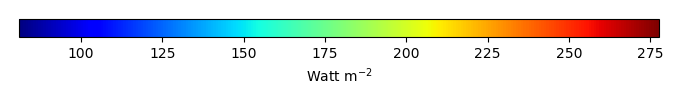MODEL MEANBIAS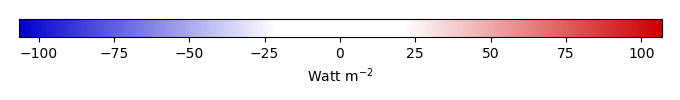BIAS SCORERMSE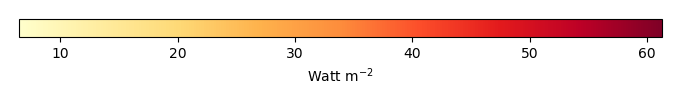RMSE SCOREBENCHMARK INTERANNUAL VARIABILITY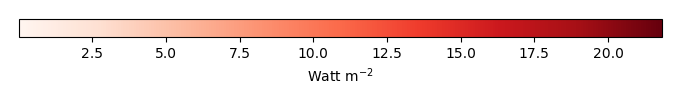MODEL INTERANNUAL VARIABILITYINTERANNUAL VARIABILITY SCOREBENCHMARK MAX MONTHMODEL MAX MONTHDIFFERENCE IN MAX MONTH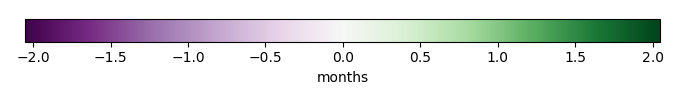SEASONAL CYCLE SCORESPATIAL TAYLOR DIAGRAMMODEL COLORS# Spatially integrated regional mean

MODEL COLORSREGIONAL MEANANNUAL CYCLEMONTHLY ANOMALYANNUAL CYCLE# All Models

BenchmarkCRUNCEPv7GSWP3v1WFDEI# Data Information

creation_date: Mon Jun 30 23:16:59 PDT 2014

source_file: This product is generated from monthly 1 degree GEWEX SRB Radiation observations